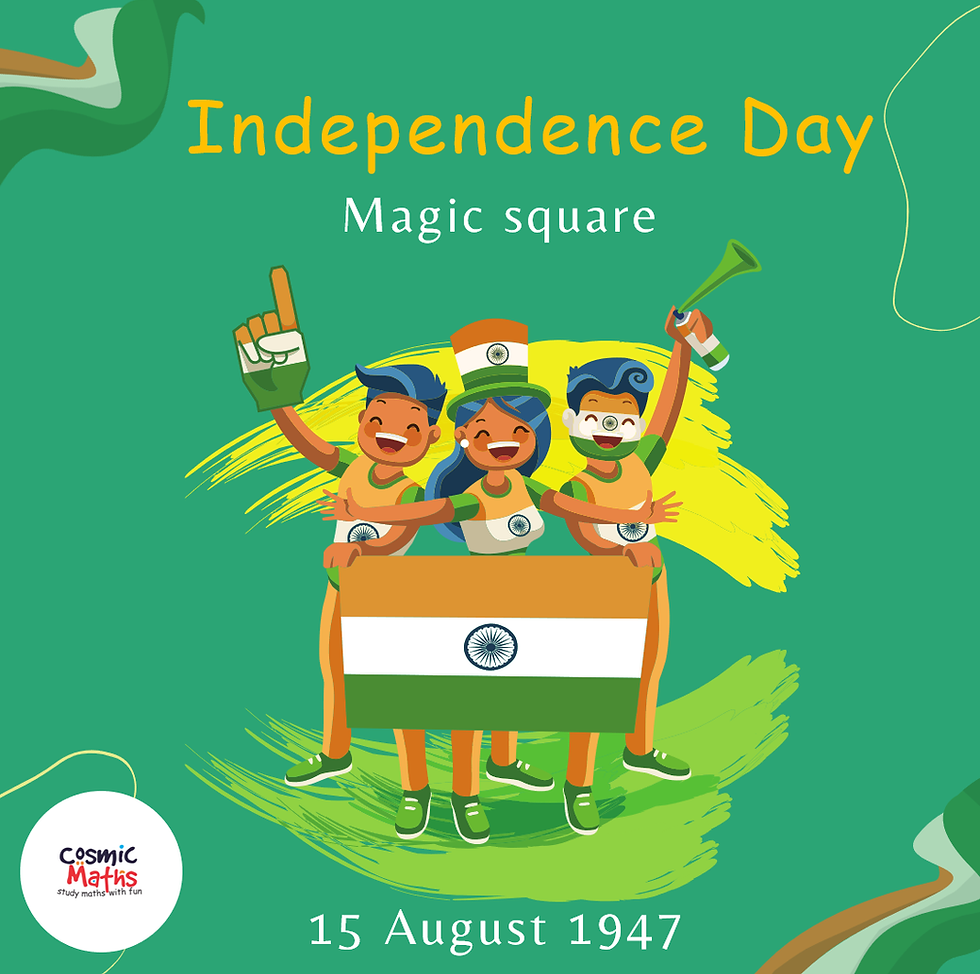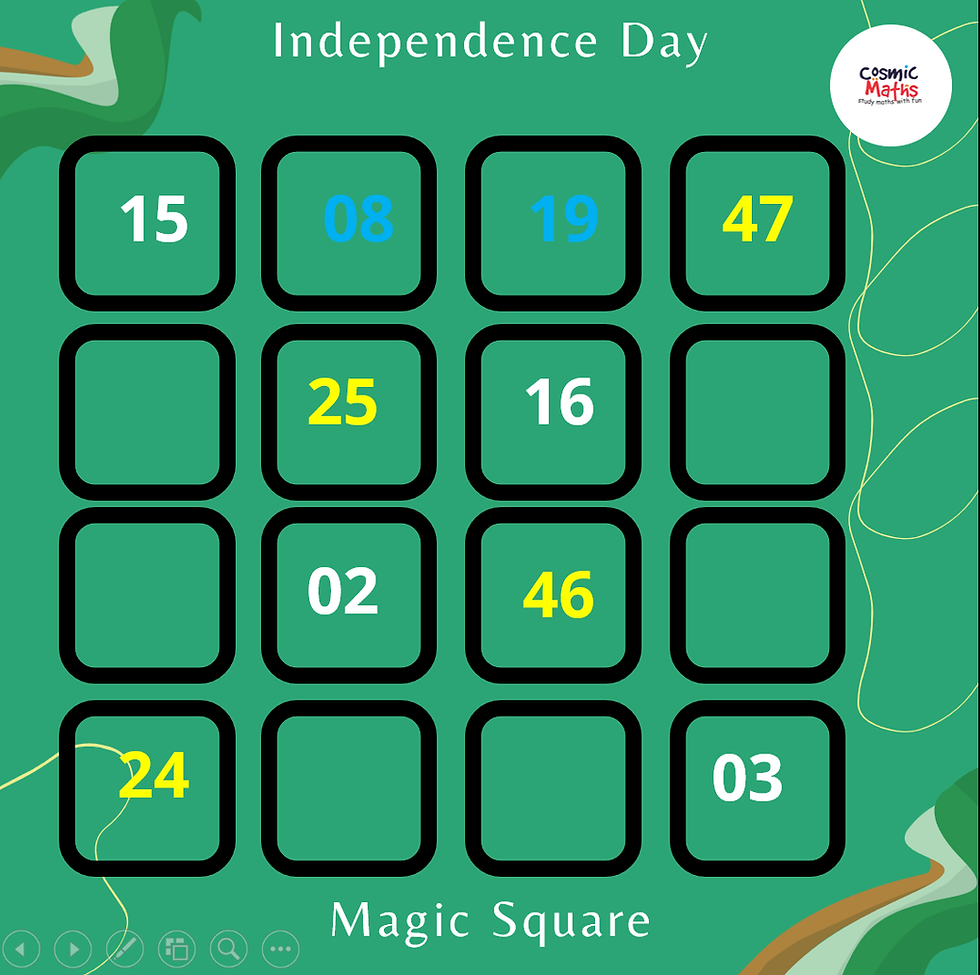top of page
Search

# Magic Square

Create Independence Day Magic SquareMagic square is a Square matrix of different numbers

Its Row totals, Column total and Diagonal totals are the same. The common total is called Magic sum.

Interest in magic squares was evinced by ancient Chinese and Indians. Narayana Pandita of the 14th century has dealt extensively with magic squares in his Ganita Kaumudi. The chapter in Ramanujan’s note book is on Magic squares. Famous mathematician Euler (1707 -83 ) also devoted a lot of his attention to magic squares.

We can construct a 4 x 4 Magic square by taking numbers from 15 Aug 19 47

•15 + 08 + 19 + 47 = 89

•08 + 19 = 27 ,Decompose/ split 27 into two numbers, ie: 24 + 3 Fill end cells of 4th rowNow end numbers of the two diagonals are available.

•End sum of one diagonal should be equal to the mid sum of the other diagonals

•Find the end sum of diagonals (15 + 3) and (24 +47) ,decompose/ split this into two numbers and insert in mid cells of the other diagonal

•: ie: 15 + 3 = 18 ; 2 +16 And 24 +47 = 71 ; 25 + 46

•Verify the Diagonal sum

15 +25 + 46 + 3 = 24 + 2 + 16 +47 =89 Magic sum.Now Middle sum of 3rd row is 2 + 46 = 48, split it to 2 numbers 30 +18 and fill the end cells of second row with these numbers.Middle sum of second row is (25 + 16) = 41 split it to 2 numbers (20 + 21 )

89 – ( 15 + 30 + 24 ) = 20

Fill the end cells of 3rd row accordingly.Middle cells of fourth row can be easily filled by using mid-end rule of symmetry

15 + 47 = 62 ; 54 + 8

89- (8 +25 + 2) =54 ; 62 – 54 = 8Check rows total, column total gives the Magic sum

Since you can split the numbers differently you can make different magic squares

Apart from getting the thrill, a positive attitude and growth mind set can be created in student, when they manage to construct a magic square.

Create your own magic squares for Independence day and share to your friends. Also you create Magic squares for your birth day Or create birth day wishes for your friend with Birth day Magic square

Wish you a happy Independence Day.

P Devaraj

cosmicmaths@gmail.com, +91 9847205803

Watch out : https://youtu.be/uyOYXAFYxbQ